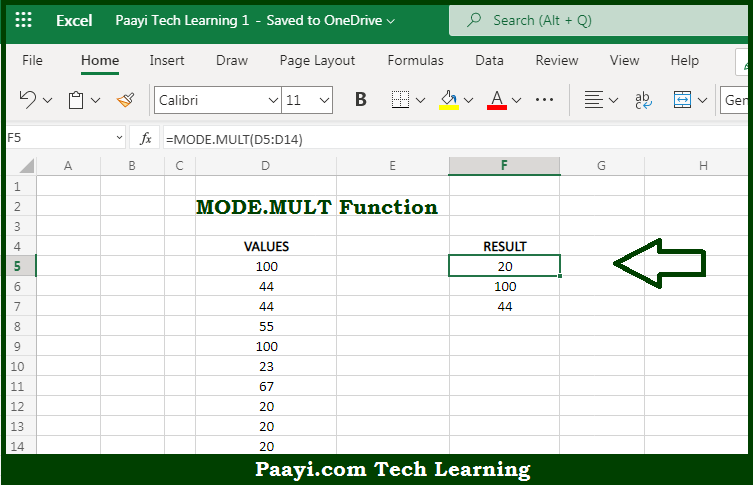# Learn How to Use Microsoft Excel MODE.MULT Function

Written by | 0 Comments | 618 Views

In this article, you will learn how to use the Microsoft Excel MODE.MULT function and its prime function in Microsoft Excel. You will also get to know the Microsoft Excel MODE.MULT function return value and syntax with the help of some examples.

Microsoft Excel MODE.MULT Function

The main purpose of the Microsoft Excel MODE.MULT function is to return the most frequently occurring numbers. That implies, with the help of MODE.MULT function you can able to return the most frequently occurring numbers in a numeric data set provided. Let's say, for example, =MODE.MULT(1,2,4,4,4,4,5,5,5,6,6,6,6) will return {4,6} as there are two modes 4 and 6. So, with the help of MODE.MULT function, you can able to get the numbers which have occurred most of the time in the numeric data set.

Return Value of MODE.MULT Function

The return value will be the number representing the mode(s).

Syntax of MODE.MULT Function

=MODE.MULT(number1, [number2], ...)

Where the arguments:

• number1: This is the number or cell reference that refers to numeric values.
• number2: This is the second number or cell reference that refers to numeric values (optional).

## How to Use Microsoft Excel MODE.MULT Function?So we know that, Microsoft Excel MODE.MULT function you can able to return the most frequently occurring numbers. That implies, with the help of MODE.MULT function you can able to return the most frequently occurring numbers in a numeric data set provided. Let's say, for example, =MODE.MULT(1,2,4,4,4,4,5,5,5,6,6,6,6) will return {4,6} as there are two modes 4 and 6. So, with the help of MODE.MULT function, you can able to get the numbers that have occurred most of the time in the numeric data set.